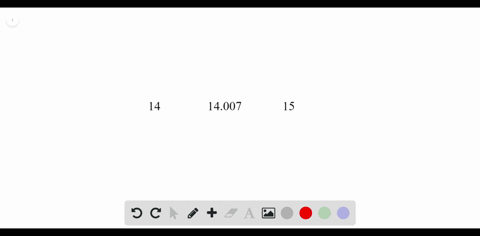### Challenge Nitrogen has two naturally occurring is…

00:35University of Miami
Problem 18

Boron (B) has two naturally occurring isotopes: boron-10 (abundance $=19.8 \%$
mass $=10.013$ amu) and boron-11 (abundance $=80.2 \%,$ mass $=11.009$ amu).
Calculate the atomic mass of boron.

10.812 amu

## Discussion

You must be signed in to discuss.

## Video Transcript

So now we'll work on problem 18 from chapter four on this problem, uh, were asked to give calculate the atomic mass of boron, given the data relating to the mass of boron 10 and 11 the mass and abundance of these. So if we're going to calculate average atomic mass for element, we need to know the exact mass of the common isotopes as well as their abundance. And so to do that, we multiply the abundance. So normally we haven't percentage form, but well, we convert to decibel so 19.8 becomes 0.198 Multiply that abundance times the mass of this particular eyes. It hope in this case here is boron 10 and then we add that to all other isotopes. So the other isotope is born 11 which has an abundance of 80.2%. So we convert that to a desperate by dividing by 100 we get 0.802 We multiply that by the mess of this isotope, which is 11.9 This is for boron 11. And when we do this multiplication and add together yet an atomic massive 10.81 a. M. You, which is our, uh, average atomic mass